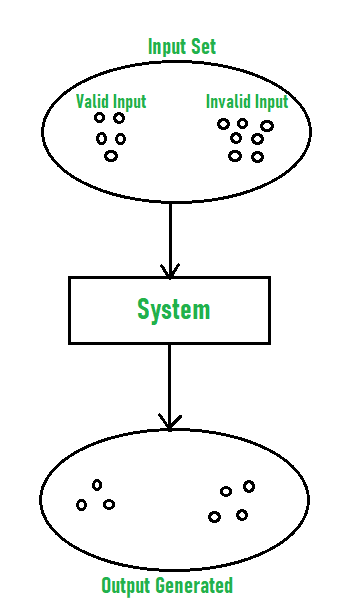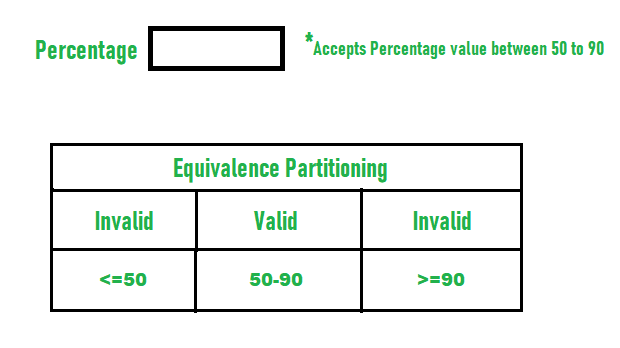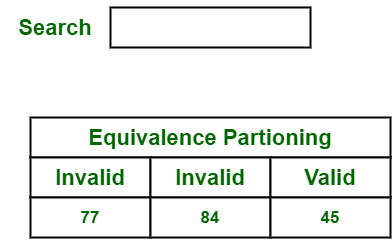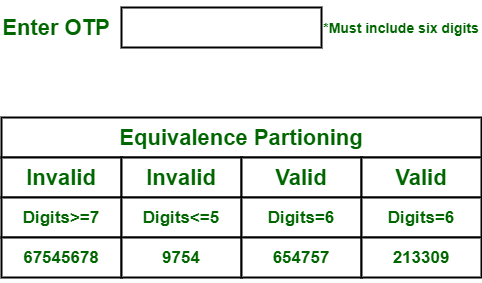# Equivalence Partitioning Method

• Difficulty Level : Easy
• Last Updated : 24 Nov, 2021

Equivalence Partitioning Method is also known as Equivalence class partitioning (ECP). It is a software testing technique or black-box testing that divides input domain into classes of data, and with the help of these classes of data, test cases can be derived. An ideal test case identifies class of error that might require many arbitrary test cases to be executed before general error is observed.

In equivalence partitioning, equivalence classes are evaluated for given input conditions. Whenever any input is given, then type of input condition is checked, then for this input conditions, Equivalence class represents or describes set of valid or invalid states.

Guidelines for Equivalence Partitioning :

• If the range condition is given as an input, then one valid and two invalid equivalence classes are defined.
• If a specific value is given as input, then one valid and two invalid equivalence classes are defined.
• If a member of set is given as an input, then one valid and one invalid equivalence class is defined.
• If Boolean no. is given as an input condition, then one valid and one invalid equivalence class is defined.Example-1:
Let us consider an example of any college admission process. There is a college that gives admissions to students based upon their percentage.

Consider percentage field that will accept percentage only between 50 to 90 %, more and even less than not be accepted, and application will redirect user to an error page. If percentage entered by user is less than 50 %or more than 90 %, that equivalence partitioning method will show an invalid percentage. If percentage entered is between 50 to 90 %, then equivalence partitioning method will show valid percentage.Example 2:
Let us consider an example of an online shopping site. In this site, each of products has a specific product ID and product name. We can search for product either by using name of product or by product ID. Here, we consider search field that accepts only valid product ID or product name.

Let us consider a set of products with product IDs and users wants to search for Mobiles. Below is a table of some products with their product Id.

If the product ID entered by user is invalid then application will redirect customer or user to error page. If product ID entered by user is valid i.e. 45 for mobile, then equivalence partitioning method will show a valid product ID.Example-3 :
Let us consider an example of software application. There is function of software application that accepts only particular number of digits, not even greater or less than that particular number.

Consider an OTP number that contains only 6 digit number, greater and even less than six digits will not be accepted, and the application will redirect customer or user to error page. If password entered by user is less or more than six characters, that equivalence partitioning method will show an invalid OTP. If password entered is exactly six characters, then equivalence partitioning method will show valid OTP.My Personal Notes arrow_drop_up